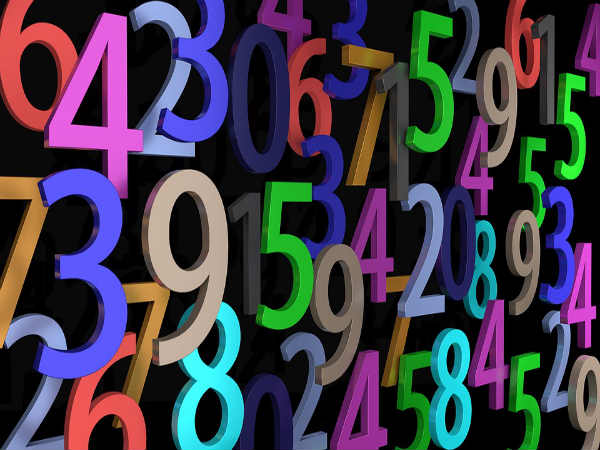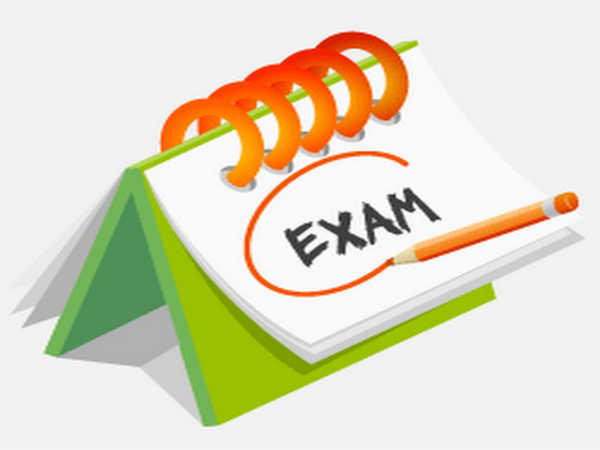Important Questions To Study For Karnataka II PUC Mathematics Exam

By Malavikka

Important Questions To Study For Karnataka II PUC Mathematics Exam

There is hardly 10 more days left for the Karnataka II PUC mathematics exam that is on Mar 12, 2018. Now is the time for students to practise a lot of previous years' question papers and important sums that are likely to appear in the 2018 board exam question paper.

CareerIndia presents to you, important marks-wise questions for Kanataka II PUC mathematics exam 2018. Go through them and revise well for the public exam. All the best!Karnataka II PUC Mathematics Exam Pattern And Weightage Scheme

Important Topics To Study

1. Types of relations

2. Principal value branches

3. Invertible matrices

4. Determinant of a square matrix

5. Derivatives of composite functions

6. Tangents

7. Integrals

8. Algebra of vectors

9. Types of LPP

10. Probability distribution of variables

Important 1 Mark Questions

1. Find the principal value of cosec ^ -1 (-root 2).

2. If A is a square matrix with |A| = 8, then find the value of |AA'|.

3. Define collinear vectors.

4. Find the direct cosines of a line which makes equal angles with the positive coordinate axes.

Important 2 Marks Questions

1. Find the values of k, if area of triangle is 4 sq cm. Units and vertices are (k,0), (4,0) and (0,2) using determinant.

2. Find the approximate change in the volume of a cube of size x metres caused by increasing the side by 3%.

3. Verification of Rolle's theorem for various functions provided.

4. Find the probability distribution of number of heads in two tosses of a coin.Karnataka 2nd PUC Exam 2018 Timetable Published: Download Now!

Important 3 Marks Questions

1. Show that the relation R in A (set of real numbers) is defined as R = [(a,b):a<=b] is reflexive and transitive but not symmetric.

2. Form the differential equation of the family of circles having centre on y axis and radius 3 units.

3. If A and B are symmetric matrices of the same order, then show that AB is symmetric if and only if AB = BA.

4. Given that the two numbers appearing on throwing two dice are different. Find the probability of the event 'the sum of numbers on the dice is 4'.

Important 5 Marks Questions

1. Let f:N->R be a function defines as f(x) = 4x^2 + 12x + 15. Show that f:N-> S, where S is the range pf f, is invertible. Find the inverse of f.

2. The length x of a rectangle is decreasing at the rate of 5cm/minute and the width y is increasing at the rate of 4cm/minute. Whe x = 8cm and y=6cm, find the rate of change of the perimeter and the area of the rectangle.

3. Derive the equation of a plane perpendicular to a given vector and passing through a given point both in vector and Cartesian form.How To Score More Than 90 In Maths For CBSE Class 12 Board Exam?

Important 10 Marks Questions

1. Show that | x x^2 yz

y y^2 zx

z z^2 xy| = (x-y)(y-z)(z-x)(xy+yz+zx)

2. Minimise and maximise z = 600x + 400y

Subject to the constraints:

x+2y<=12

2x+y<=12

4x+5y>=20 and x>=0, y>=0 by graphical method.

Subscribe Now

--Or--
Select a Field of Study
Select a Course
Select UPSC Exam
Select IBPS Exam
Select Entrance Exam